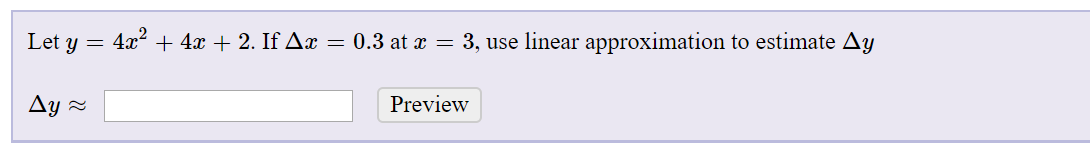# Let y = 4x?+ 4x + 2. If Ax = 0.3 at x = 3, use linear approximation to estimate AyAy =Preview

Question
2 viewshelp_outlineImage TranscriptioncloseLet y = 4x? + 4x + 2. If Ax = 0.3 at x = 3, use linear approximation to estimate Ay Ay = Preview fullscreen
check_circle

Step 1

Given:

Step 2

Concept:

For linear approximation:

Step 3

The required values a...

### Want to see the full answer?

See Solution

#### Want to see this answer and more?

Solutions are written by subject experts who are available 24/7. Questions are typically answered within 1 hour.*

See Solution
*Response times may vary by subject and question.
Tagged in

### Calculus